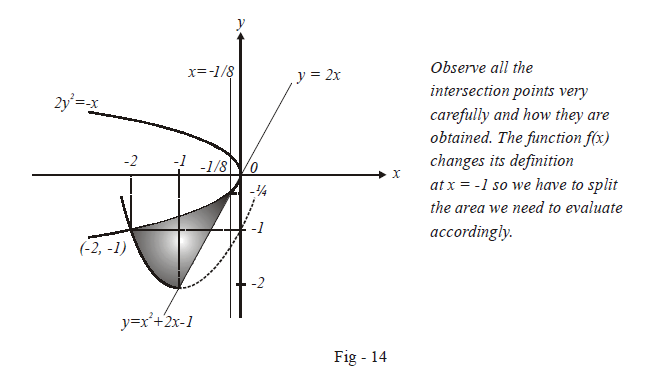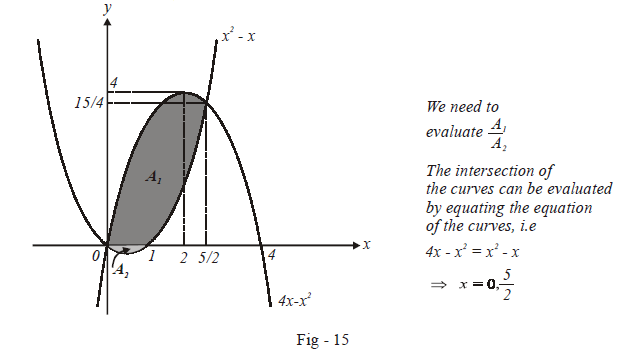# Problems On Areas Set-7

Go back to  'Areas and Volumes'

Example – 13

Let $$f\left( x \right)$$ be a continuous function given by

$f\left( x \right) = \left\{ \begin{array}{l}2x,{\rm{ }}\left| x \right| \le 1\\{x^2} + ax + b{\rm{ }}\left| x \right| > 1\end{array} \right\}$

Find the area of the region in the third quadrant bounded by the curves $$x = - 2{y^2}\,{\rm{and}}\,{\rm{ }}y = f(x)$$ lying to the left of the line $$8x + 1 = 0$$ .

Solution: We can use the fact that $$f\left( x \right)$$ is continuous to determine the constants $$a$$ and $$b.$$ The critical points are $$x = {\rm{ }}-1{\rm{ }}\;\text{and}\;x = {\rm{ }}1$$ .

$$\fbox{x = 1}$$

\begin{align}& \qquad \mathop {\lim }\limits_{x \to - {1^ - }} f(x) = f( - 1)\\& \Rightarrow \quad 1 - a + b = - 2\\& \Rightarrow \quad a - b = 3 \qquad \qquad \dots (1)\end{align}

$$\fbox{x = -1}$$

\begin{align}&\qquad \mathop {\lim }\limits_{x \to {1^ + }} f(x) = f(1)\\&\Rightarrow \quad 1 + a + b = 2\\&\Rightarrow \quad a + b = 1 \qquad \qquad \dots (2)\end{align}

From (1) and (2) $$a = 2 \; \text{and} \; b = - 1$$ ,

Thus,

$f(x) = \left\{ \begin{array}{l}2x,{\rm{ }}\left| x \right| \le 1\\{x^2} + 2x - 1,{\rm{ }}\left| x \right| > 1\end{array} \right\}$

We now proceed to sketch the bounded region that has been described in the question.The required area is:

\begin{align}A&= \int\limits_{ - 2}^{ - 1} {\left( {\sqrt {\frac{{ - x}}{2}} - ({x^2} + 2x - 1)} \right)} dx + \int\limits_{ - 1}^{ - 1/8} {\left( {\sqrt { - \frac{x}{2}} - 2x} \right)} dx \\\\&= \left. {\left\{ { - \frac{{\sqrt 2 }}{3}{{( - x)}^{3/2}} - \left( {\frac{{{x^3}}}{3} + {x^2} - x} \right)} \right\}} \right|_{ - 2}^{ - 1} + \left. {\left\{ {\frac{{ - \sqrt 2 }}{3}{{( - x)}^{3/2}} - {x^2}} \right\}} \right|_{ - 1}^{ - 1/8}\\\\ & = \left\{ { - \frac{{\sqrt 2 }}{3}(1 - {2^{3/2}}) - \left( {\frac{5}{3} - \frac{{10}}{3}} \right)} \right\} + \left\{ {\frac{{ - \sqrt 2 }}{3}\left( {\frac{1}{{{8^{3/2}}}} - 1} \right) - \left( {\frac{1}{{64}} - 1} \right)} \right\}\\\\&= - \frac{{\sqrt 2 }}{3} + \frac{4}{3} + \frac{5}{3} - \frac{1}{{48}} + \frac{{\sqrt 2 }}{3} - \frac{1}{{64}} + 1\\\\&= - \frac{1}{{48}} - \frac{1}{{64}}\\\\& = 4 - \frac{7}{{192}}\\\\&= \frac{{761}}{{192}}{\rm{sq}}{\rm{. units}}\end{align}

Example – 14

In what ratio does the $$x-$$axis divide the area of the region bounded by the parabola $${\rm{y = 4x - }}{{\rm{x}}^2}{\rm{ \;and \; y = }}{{\rm{x}}^2}{\rm{ - x ?}}$$

Solution: The two parabolas and the region they bound have been sketched below:The total area $${A_1} + {A_2}$$ can be evaluated as the area of the region bounded between the two curves:

\begin{align}{A_1} + {A_2}&= \int\limits_0^{5/2} {\left\{ {(4x - {x^2}) - ({x^2} - x)} \right\}} dx \\& = \int\limits_0^{5/2} {(5x - 2{x^2})} dx\\&= \left. {\left( {\frac{{5{x^2}}}{2} - \frac{{2{x^3}}}{3}} \right)} \right|_0^{5/2}\\&= \frac{{125}}{8} - \frac{{125}}{{12}}\\&= \frac{{125}}{{24}}{\rm{sq}}{\rm{. units}}\end{align}

The area $${A_2}$$ is

\begin{align}{A_2}&= \left| {\int\limits_0^1 {\left( {{x^2} - x} \right)dx} } \right|\\\\&= \left| {\left. {\left( {\frac{{{x^3}}}{3} - \frac{{{x^2}}}{2}} \right)} \right|_0^1} \right|\\\\&= \frac{1}{6}{\rm{sq}}{\rm{. units}} \end{align}

The area $${A_1}$$ is therefore

\begin{align}{A_1}&= \frac{{125}}{{24}} - \frac{1}{6}\\\\&= \frac{{121}}{{24}}{\rm{sq}}{\rm{. units}}\end{align}

Thus, the required ratio is

$\frac{{{A_1}}}{{{A_2}}} = \frac{{121}}{4}$